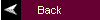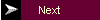MATLAB Tutorial2. Fourier Analysis

Commands covered:

dft
idft
fft
ifft
contfft

The dft command uses a straightforward method to compute the discrete Fourier transform. Define a vector x and compute the DFT using the command

X = dft(x)

The first element in X corresponds to the value of X(0).

The command idft uses a straightforward method to compute the inverse discrete Fourier transform. Define a vector X and compute the IDFT using the command

x = idft(X)

The first element of the resulting vector x is x.

For a more efficient but less obvious program, the discrete Fourier transform can be computed using the command fft which performs a Fast Fourier Transform of a sequence of numbers. To compute the FFT of a sequence x[n] which is stored in the vector x, use the command

X = fft(x)

Used in this way, the command fft is interchangeable with the command dft. For more computational efficiency, the length of the vector x should be equal to an exponent of 2, that is 64, 128, 512, 1024, 2048, etc. The vector x can be padded with zeros to make it have an appropriate length. MATLAB does this automatically by using the following command where N is defined to be an exponent of 2:

X = fft(x,N);

The longer the length of x, the finer the grid will be for the FFT. Due to a wrap around effect, only the first N/2 points of the FFT have any meaning.

The ifft command computes the inverse Fourier transform:

x = ifft(X);

The FFT can be used to approximate the Fourier transform of a continuous-time signal as shown in Section 6.6 of the textbook. A continuous-time signal x(t) is sampled with a period of T seconds, then the DFT is computed for the sampled signal. The resulting amplitude must be scaled and the corresponding frequency determined. An M-file that approximates the Fourier Transform of a sampled continuous-time signal can be downloaded from contfft.m. Let a vector x be defined as the sampled continuous-time signal x(t) and let T be the sampling time.

[X,w] = contfft(x,T);

The outputs are the Fourier transform stored in the vector X and the corresponding frequency vector w.

This tutorial is available as a supplement to the textbook Fundamentals of Signals and Systems Using Matlab by Edward Kamen and Bonnie Heck, published by Prentice Hall. The tutorial is designed for students using either the professional version of MATLAB (ver. 5.0) with the Control Systems Toolbox (ver. 4.0) and the Signal Processing Toolbox (ver. 4.0), or using the Student Edition of MATLAB (ver. 5.0). For more information on MATLAB, contact The Mathworks, Inc.